# IBDP Maths AI: Topic : SL 1.8: Systems of linear equations: IB style Questions SL Paper 1

### Question

2. The ticket prices for a concert are shown in the following table.

 Ticket Type Price (in Australian dollars, $) Adut 15 Child 10 Student 12 • A total of 600 tickets were sold. • The total amount of money from ticket sales was$7816.
• There were twice as many adult tickets sold as child tickets.

Let the number of adult tickets sold be x , the number of child tickets sold be y , and the
number of student tickets sold be z.

(a) Write down three equations that express the information given above.
(b) Find the number of each type of ticket sold.

Ans:

(a) x + y + z = 600
15x + 10y + 12z = 7816
x = 2y

(b) x = 308, y = 154, z = 138

### Question

5. The ticket prices for a concert are shown in the following table.

 Ticket Type Price (in Australian dollar, $) Adult 15 Child 10 Student 12 • A total of 600 tickets were sold. • The total amount of money from ticket sales was$7816.
• There were twice as many adult tickets sold as child tickets

Let the number of adult tickets sold be x , the number of child tickets sold be y , and the
number of student tickets sold be z.
(a) Write down three equations that express the information given above.
(b) Find the number of each type of ticket sold.

Ans:

(a) x + y + z = 600
15x + 10y + 12z = 7816
x = 2y
(b) x = 308, y = 154, z = 138

## Question

A store sells bread and milk. On Tuesday, 8 loaves of bread and 5 litres of milk were sold for $21.40. On Thursday, 6 loaves of bread and 9 litres of milk were sold for$23.40.

If $$b =$$ the price of a loaf of bread and $$m =$$ the price of one litre of milk, Tuesday’s sales can be written as $$8b + 5m = 21.40$$.

Using simplest terms, write an equation in b and m for Thursday’s sales.

a.

Find b and m.

b.

Draw a sketch, in the space provided, to show how the prices can be found graphically.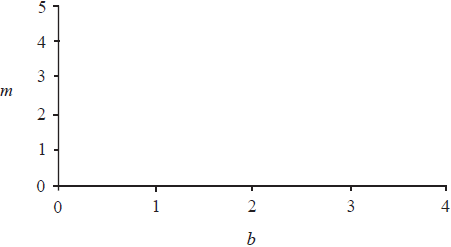c.

## Markscheme

Thursday’s sales, $$6b + 9m = 23.40$$     (A1)

$$2b + 3m = 7.80$$     (A1)     (C2)[2 marks]

a.

$$m = 1.40$$     (accept 1.4)     (A1)(ft)

$$b = 1.80$$     (accept 1.8)     (A1)(ft)

Award (A1)(d) for a reasonable attempt to solve by hand and answer incorrect.     (C2)[2 marks]

b.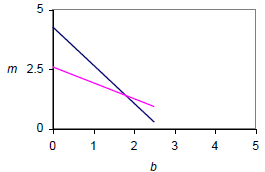(A1)(A1)(ft)

(A1) each for two reasonable straight lines. The intersection point must be approximately correct to earn both marks, otherwise penalise at least one line.

Note: The follow through mark is for candidate’s line from (a).     (C2)[2 marks]

c.

## Question

The number of cells, C, in a culture is given by the equation $$C = p \times 2^{0.5t} + q$$, where t is the time in hours measured from 12:00 on Monday and p and q are constants.

The number of cells in the culture at 12:00 on Monday is 47.

The number of cells in the culture at 16:00 on Monday is 53.

Use the above information to write down two equations in p and q ;

a.

Use the above information to calculate the value of p and of q ;

b.

Use the above information to find the number of cells in the culture at 22:00 on Monday.

c.

## Markscheme

p + q = 47     (A1)

4p + q = 53     (A1)     (C2)[2 marks]

a.

Reasonable attempt to solve their equations     (M1)

p = 2, q = 45     (A1)     (C2)

Note: Accept only the answers p = 2, q = 45.[2 marks]

b.

C = 2 × 20.5(10) + 45     (M1)

C = 109     (A1)(ft)     (C2)

Note: Award (M1) for substitution of 10 into the formula with their values of p and q.[2 marks]

c.

## Question

Consider the function $$f(x) = p{(0.5)^x} + q$$ where p and q are constants. The graph of f (x) passes through the points $$(0,\,6)$$ and $$(1,\,4)$$ and is shown below.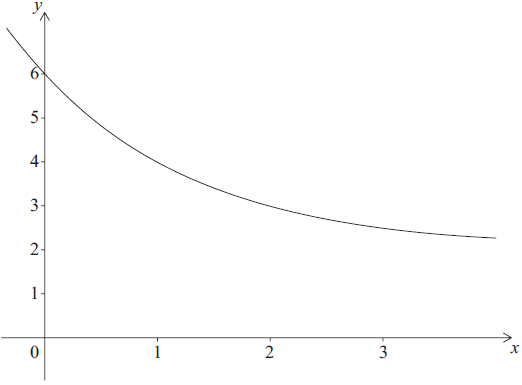Write down two equations relating p and q.

a.

Find the value of p and of q.

b.

Write down the equation of the horizontal asymptote to the graph of f (x).

c.

## Markscheme

p + q = 6     (A1)

0.5p + q = 4     (A1)     (C2)

Note: Accept correct equivalent forms of the equations.[2 marks]

a.

p = 4, q = 2     (A1)(A1)(ft)     (C2)

Notes: If both answers are incorrect, award (M1) for attempt at solving simultaneous equations.[2 marks]

b.

y = 2     (A1)(A1)(ft)     (C2)

Notes: Award (A1) for “y = a constant”, (A1)(ft) for 2. Follow through from their value for q as long as their constant is greater than 2 and less than 6.

An equation must be seen for any marks to be awarded.[2 marks]

c.

## Question

$$10 000$$ people attended a sports match. Let $$x$$ be the number of adults attending the sports match and $$y$$ be the number of children attending the sports match.

Write down an equation in $$x$$ and $$y$$ .

a.

The cost of an adult ticket was $$12$$ Australian dollars (AUD). The cost of a child ticket was $$5$$ Australian dollars (AUD).

Find the total cost for a family of 2 adults and 3 children.

b.

The total cost of tickets sold for the sports match was $$108800{\text{ AUD}}$$.

Write down a second equation in $$x$$ and $$y$$ .

c.

Write down the value of $$x$$ and the value of $$y$$ .

d.

## Markscheme

$$x + y = 10000$$     (A1)     (C1)[1 mark]

a.

$$2 \times 12 + 3 \times 5$$     (M1)

$$39{\text{ }}(39.0{\text{, }}39.00)$$ (AUD)     (A1)     (C2)[2 marks]

b.

$$12x + 5y = 108800$$     (A1)     (C1)[1 mark]

c.

$$x = 8400$$, $$y = 1600$$     (A1)(ft)(A1)(ft)     (C2)

Notes: Follow through from their equations. If $$x$$ and $$y$$ are both incorrect then award (M1) for attempting to solve simultaneous equations.[2 marks]

d.

## Question

A function f (x) = p×2x + q is defined by the mapping diagram below.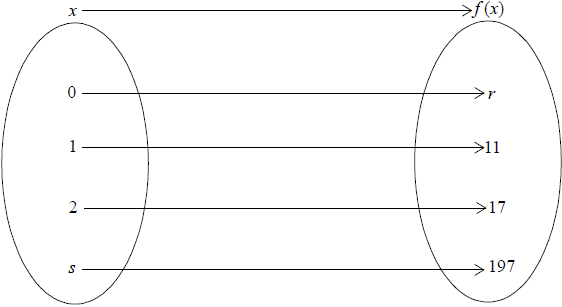Find the value of

(i) p ;

(ii) q .

a.

Write down the value of r .

b.

Find the value of s .

c.

## Markscheme

(i) 2p + q = 11 and 4p + q = 17     (M1)

Note: Award (M1) for either two correct equations or a correct equation in one unknown equivalent to 2p = 6 .

p = 3     (A1)

(ii) q = 5     (A1)     (C3)

Notes: If only one value of p and q is correct and no working shown, award (M0)(A1)(A0).[3 marks]

a.

r = 8     (A1)(ft)     (C1)

Note: Follow through from their answers for p and q irrespective of whether working is seen.[1 mark]

b.

3 × 2s + 5 = 197     (M1)

Note: Award (M1) for setting the correct equation.

s = 6     (A1)(ft)     (C2)

Note: Follow through from their values of p and q.[2 marks]

c.

## Question

The equation of a line L1 is $$2x + 5y = −4$$.

Write down the gradient of the line L1.

a.

A second line L2 is perpendicular to L1.

Write down the gradient of L2.

b.

The point (5, 3) is on L2.

Determine the equation of L2.

c.

Lines L1 and L2 intersect at point P.

Using your graphic display calculator or otherwise, find the coordinates of P.

d.

## Markscheme

$$\frac{-2}{{5}}$$     (A1)     (C1)

a.

$$\frac{5}{{2}}$$     (A1)(ft)     (C1)

b.

$$3 = \frac{5}{2} \times 5 + c$$     (M1)

Notes: Award (M1) for correct substitution of their gradient into equation of line. Follow through from their answer to part (b).

$$y = \frac{5}{2}x – \frac{19}{2}$$     (A1)(ft)

OR

$$y – 3 = \frac{5}{2}(x – 5)$$     (M1)(A1)(ft)     (C2)

Notes: Award (M1) for correct substitution of their gradient into equation of line. Follow through from their answer to part (b).

c.

(3, −2)     (A1)(ft)(A1)(ft)     (C2)

Notes: If parentheses not seen award at most (A0)(A1)(ft). Accept x = 3, y = −2. Follow through from their answer to part (c), even if no working is seen. Award (M1)(A1)(ft) for a sensible attempt to solve $$2x + 5y = −4$$ and their $$y = \frac{5}{2}x – \frac{19}{2}$$ or equivalent, simultaneously.

d.

## Question

A quadratic function $$f:x \mapsto a{x^2} + b$$, where $$a$$ and $$b \in \mathbb{R}$$ and $$x \geqslant 0$$, is represented by the mapping diagram.Using the mapping diagram, write down two equations in terms of $$a$$ and $$b$$.

a.

Solve the equations to find the value of

(i)     $$a$$;

(ii)     $$b$$.

b.

Find the value of $$c$$.

c.

## Markscheme

$$a{(1)^2} + b = – 9$$     (A1)

$$a{(3)^2} + b = 119$$     (A1)     (C2)

Note: Accept equivalent forms of the equations.[2 marks]

a.

(i)     $$a = 16$$     (A1)(ft)

(ii)     $$b = – 25$$     (A1)(ft)     (C2)

Note: Follow through from part (a) irrespective of whether working is seen.

If working is seen follow through from part (i) to part (ii).[2 marks]

b.

$$16{c^2} – 25 = 171$$     (M1)

Note: Award (M1) for correct quadratic with their $$a$$ and $$b$$ substituted.

$$c = 3.5$$     (A1)(ft)     (C2)

Note: Accept $$x$$ instead of $$c$$.

Award (A1) only, for an answer of $$\pm 3.5$$ with or without working.[2 marks]

c.

## Question

The graph of the quadratic function $$f(x) = a{x^2} + bx + c$$ intersects the y-axis at point A (0, 5) and has its vertex at point B (4, 13).Write down the value of $$c$$.

a.

By using the coordinates of the vertex, B, or otherwise, write down two equations in $$a$$ and $$b$$.

b.

Find the value of $$a$$ and of $$b$$.

c.

## Markscheme

5     (A1)     (C1)[1 mark]

a.

at least one of the following equations required

$$a{(4)^2} + 4b + 5 = 13$$

$$4 = – \frac{b}{{2a}}$$

$$a{(8)^2} + 8b + 5 = 5$$     (A2)(A1)     (C3)

Note: Award (A2)(A0) for one correct equation, or its equivalent, and (C3) for any two correct equations.

The equation $$a{(0)^2} + b(0) = 5$$ earns no marks.[3 marks]

b.

$$a = – \frac{1}{2},{\text{ }}b = 4$$     (A1)(ft)(A1)(ft)     (C2)

Note: Follow through from their equations in part (b), but only if their equations lead to unique solutions for $$a$$ and $$b$$.[2 marks]

c.

## Question

An iron bar is heated. Its length, $$L$$, in millimetres can be modelled by a linear function, $$L = mT + c$$, where $$T$$ is the temperature measured in degrees Celsius (°C).

At 150°C the length of the iron bar is 180 mm.

Write down an equation that shows this information.

a.

At 210°C the length of the iron bar is 181.5 mm.

Write down an equation that shows this second piece of information.

b.

At 210°C the length of the iron bar is 181.5 mm.

Hence, find the length of the iron bar at 40°C.

c.

## Markscheme

$$180 = 150m + c\;\;\;$$(or equivalent)     (A1)     (C1)

a.

$$181.5 = 210m + c\;\;\;$$(or equivalent)     (A1)     (C1)

b.

$$m = 0.25,{\text{ }}c = 176.25$$     (A1)(A1)(ft)

Note: Follow through from part (a) and part (b), irrespective of working shown.

$$L = 0.025(4) + 176.25$$     (M1)

Note: Award (M1) for substitution of their $$m$$, their $$c$$ and 40 into equation.

$$L = 177\;\;\;(177.25){\text{ (mm)}}$$     (A1)(ft)     (C4)

Note: Follow through, within part (c), from their $$m$$ and $$c$$ only if working shown.

c.

## Question

A liquid is heated so that after $$20$$ seconds of heating its temperature, $$T$$ , is $${25^ \circ }{\text{C}}$$ and after $$50$$ seconds of heating its temperature is $${37^ \circ }{\text{C}}$$ .

The temperature of the liquid at time $$t$$ can be modelled by $$T = at + b$$ , where $$t$$ is the time in seconds after the start of heating.

Using this model one equation that can be formed is $$20a + b = 25$$ .

Using the model, write down a second equation in $$a$$ and $$b$$ .

a.

Using your graphic display calculator or otherwise, find the value of $$a$$ and of $$b$$ .

b.

Use the model to predict the temperature of the liquid $$60{\text{ seconds}}$$ after the start of heating.

c.

## Markscheme

$$50a + b = 37$$ (A1)(A1)     (C2)

Note: Award (A1) for $$50a + b$$ , (A1) for $$= 37$$ .

a.

$$a = 0.4$$, $$b = 17$$     (A1)(ft)(A1)(ft)     (C2)

Notes: Award (M1) for attempt to solve their equations if this is done analytically. If the GDC is used, award (ft) even if no working seen.

b.

$$T = 0.4(60) + 17$$     (M1)

Note: Award (M1) for correct substitution of their values and $$60$$ into equation for $$T$$.

$$T = 41{\text{ }}{{\text{(}}^ \circ }{\text{C}})$$     (A1)(ft)     (C2)

Note: Follow through from their part (b).

c.

## Question

The length of one side of a rectangle is 2 cm longer than its width.

If the smaller side is x cm, find the perimeter of the rectangle in terms of x.

a.

The length of one side of a rectangle is 2 cm longer than its width.

The perimeter of a square is equal to the perimeter of the rectangle in part (a).

Determine the length of each side of the square in terms of x.

b.

The length of one side of a rectangle is 2 cm longer than its width.

The perimeter of a square is equal to the perimeter of the rectangle in part (a).

The sum of the areas of the rectangle and the square is $$2x^2 + 4x +1$$ (cm2).

(i) Given that this sum is 49 cm2, find x.

(ii) Find the area of the square.

c.

## Markscheme

Unit penalty (UP) is applicable where indicated in the left hand column.

(UP) $${\text{P (rectangle)}} = 2x + 2(x + 2) = 4x + 4{\text{ cm}}$$     (A1)     (C1)

(UP) Simplification not required[1 mark]

a.

Unit penalty (UP) is applicable where indicated in the left hand column.

(UP) Side of square = (4x + 4)/4 = x + 1 cm     (A1)(ft)     (C1)[1 mark]

b.

(i) $$2x^2 + 4x + 1 = 49$$ or equivalent     (M1)

$$(x + 6)(x – 4) = 0$$

$$x = – 6$$ and $$4$$     (A1)

Note: award (A1) for the values or for correct factors

Choose $$x = 4$$     (A1)(ft)

Award (A1)(ft) for choosing positive value.     (C3)

(ii) $${\text{Area of square}} = 5 \times 5 = 25{\text{ c}}{{\text{m}}^2}$$     (A1)(ft)

Note: Follow through from both (b) and (c)(i).     (C1)[4 marks]

c.

## Question

Solve the following equation for x

$$3(2x +1) − 2(3 − x)=13$$.

a.

Factorize the expression $$x^2 + 2x − 3$$.

b.

Find the positive solution of the equation

$$x^2 + 2x − 6 = 0$$.

c.

## Markscheme

$$6x+ 3 – 6 + 2x = 13$$     (M1)

$$8x = 16$$

$$x = 2$$     (A1)     (C2)[2 marks]

a.

$$(x + 3) (x – 1)$$     (A1)(A1)     (C2)[2 marks]

b.

$$x = 1.64575…$$

$$x = 1.65$$     (A2)

If formula is used award (M1)(A1) for correct solution. If graph is sketched award (M1)(A1) for correct solution.     (C2)[2 marks]

c.

## Question

Factorise the expression $${x^2} – kx$$ .

a.

Hence solve the equation $${x^2} – kx = 0$$ .

b.

The diagram below shows the graph of the function $$f(x) = {x^2} – kx$$ for a particular value of $$k$$.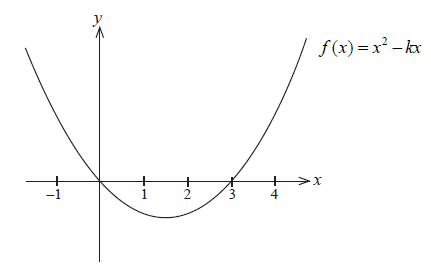Write down the value of $$k$$ for this function.

c.

The diagram below shows the graph of the function $$f(x) = {x^2} – kx$$ for a particular value of $$k$$.Find the minimum value of the function $$y = f(x)$$ .

d.

## Markscheme

$$x(x – k)$$     (A1)     (C1)[1 mark]

a.

$$x = 0$$ or $$x = k$$     (A1)     (C1)

Note: Both correct answers only.[1 mark]

b.

$$k = 3$$     (A1)     (C1)[1 mark]

c.

$${\text{Vertex at }}x = \frac{{ – ( – 3)}}{{2(1)}}$$     (M1)

Note: (M1) for correct substitution in formula.

$$x = 1.5$$     (A1)(ft)

$$y = – 2.25$$     (A1)(ft)

OR

$$f'(x) = 2x – 3$$     (M1)

Note: (M1) for correct differentiation.

$$x = 1.5$$     (A1)(ft)
$$y = – 2.25$$     (A1)(ft)

OR

for finding the midpoint of their 0 and 3     (M1)
$$x = 1.5$$     (A1)(ft)
$$y = – 2.25$$     (A1)(ft)

Note: If final answer is given as $$(1.5{\text{, }}{- 2.25})$$ award a maximum of (M1)(A1)(A0)[3 marks]

d.

## Question

Let $$f (x) = x^2 – 6x + 8$$.

Factorise $$x^2 – 6x + 8$$.

a.

Hence, or otherwise, solve the equation $$x^2 – 6x + 8 = 0$$.

b.

Let $$g(x) = x + 3$$.

Write down the solutions to the equation $$f (x) = g(x)$$.

c.

## Markscheme

$$(x – 2)(x – 4)$$     (A1)(A1)     (C2)[2 marks]

a.

x = 2, x = 4     (A1)(ft)(A1)(ft)     (C2)[2 marks]

b.

x = 0.807, x = 6.19     (A1)(A1)     (C2)

Note: Award maximum of (A0)(A1) if coordinate pairs given.

OR

(M1) for an attempt to solve $$x^2 – 7x + 5 = 0$$ via formula with correct values substituted.     (M1)

$$x = \frac{{7 \pm \sqrt {29} }}{2}$$     (A1)     (C2)[2 marks]

c.

## Question

The length of a square garden is (x + 1) m. In one of the corners a square of 1 m length is used only for grass. The rest of the garden is only for planting roses and is shaded in the diagram below.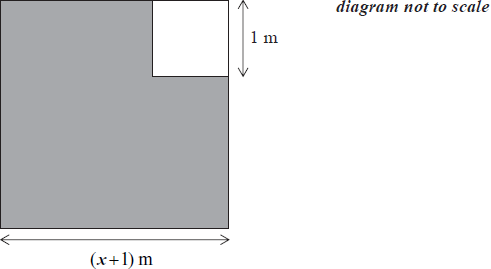The area of the shaded region is A .

Write down an expression for A in terms of x.

a.

Find the value of x given that A = 109.25 m2.

b.

The owner of the garden puts a fence around the shaded region. Find the length of this fence.

c.

## Markscheme

(x + 1)2 – 1  or  x2 + 2x     (A1)     (C1)[1 mark]

a.

(x + 1)2 – 1 = 109.25     (M1)

x2 + 2x – 109.25 = 0     (M1)

Notes: Award (M1) for writing an equation consistent with their expression in (a) (accept equivalent forms), (M1) for correctly removing the brackets.

OR

(x + 1)2 – 1 = 109.25     (M1)

x + 1 = $$\sqrt {110.25}$$     (M1)

Note: Award (M1) for writing an equation consistent with their expression in (a) (accept equivalent forms), (M1) for taking the square root of both sides.

OR

(x + 1)2 – 10.52 = 0     (M1)

(x – 9.5) (x + 11.5) = 0     (M1)

Note: Award (M1) for writing an equation consistent with their expression in (a) (accept equivalent forms), (M1) for factorised left side of the equation.

x = 9.5     (A1)(ft)     (C3)

Note: Follow through from their expression in part (a).

The last mark is lost if x is non positive.

If the follow through equation is not quadratic award at most (M1)(M0)(A1)(ft).[3 marks]

b.

4 × (9.5 + 1) = 42 m     (M1)(A1)(ft)     (C2)

Notes: Award (M1) for correct method for finding the length of the fence. Accept equivalent methods.[2 marks]

c.

## Question

In the diagram, $${\text{B}}\hat {\text{A}}{\text{C}} = {90^ \circ }$$ . The length of the three sides are $$x{\text{ cm}}$$, $$(x + 7){\text{ cm}}$$ and $$(x + 8){\text{ cm}}$$.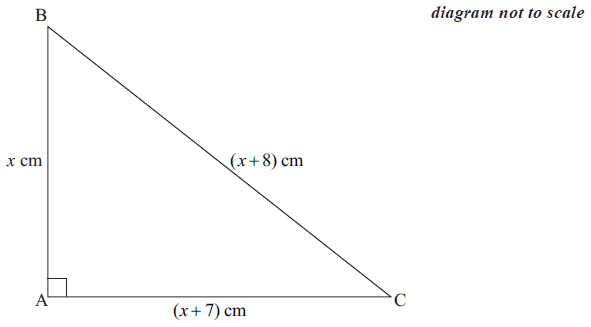Write down and simplify a quadratic equation in $$x$$ which links the three sides of the triangle.

a.

Solve the quadratic equation found in part (a).

b.

Write down the value of the perimeter of the triangle.

c.

## Markscheme

$${(x + 8)^2} = {(x + 7)^2} + {x^2}$$     (A1)

Note: Award (A1) for a correct equation.

$${x^2} + 16x + 64 = {x^2} + 14x + 49 + {x^2}$$     (A1)

Note: Award (A1) for correctly removed parentheses.

$${x^2} – 2x – 15 = 0$$     (A1)     (C3)

Note: Accept any equivalent form.[3 marks]

a.

$$x = 5$$, $$x = – 3$$     (A1)(ft)(A1)(ft)     (C2)

Notes: Accept (A1)(ft) only from the candidate’s quadratic equation.[2 marks]

b.

$$30{\text{ cm}}$$     (A1)(ft)     (C1)

Note: Follow through from a positive answer found in part (b).[1 mark]

c.

## Question

In the diagram, triangle ABC is isosceles. AB = AC and angle ACB is 32°. The length of side AC is x cm.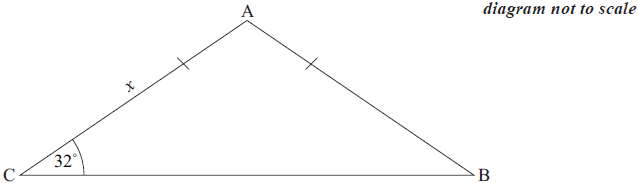Write down the size of angle CBA.


a.

Write down the size of angle CAB.

b.

The area of triangle ABC is 360 cm2. Calculate the length of side AC. Express your answer in millimetres.

c.

## Markscheme

32°     (A1)     (C1)[1 mark]

a.

116°     (A1)     (C1)[1 mark]

b.

$$360 = \frac{1}{2} \times {x^2} \times \sin 116^\circ$$     (M1)(A1)(ft)

Notes: Award (M1) for substitution into correct formula with 360 seen, (A1)(ft) for correct substitution, follow through from their answer to part (b).

x = 28.3 (cm)     (A1)(ft)

x = 283 (mm)     (A1)(ft)     (C4)

Notes: The final (A1)(ft) is for their cm answer converted to mm. If their incorrect cm answer is seen the final (A1)(ft) can be awarded for correct conversion to mm.[4 marks]

c.

## Question

f (x) = 5x3 − 4x2 + x

Find f‘(x).

a.

(i) the local maximum point;

(ii) the local minimum point.

b.

## Markscheme

15x2 – 8x + 1     (A1)(A1)(A1)     (C3)

Note: Award (A1) for each correct term.[3 marks]

a.

15x2 – 8x +1 = 0     (A1)(ft)

Note: Award (A1)(ft) for setting their derivative to zero.

(i) $$(x =)\frac{1}{5}(0.2)$$     (A1)(ft)

(ii) $$(x =)\frac{1}{3}(0.333)$$     (A1)(ft)     (C3)

b.

## Question

The graph of the quadratic function $$f (x) = c + bx − x^2$$ intersects the y-axis at point A(0, 5) and has its vertex at point B(2, 9).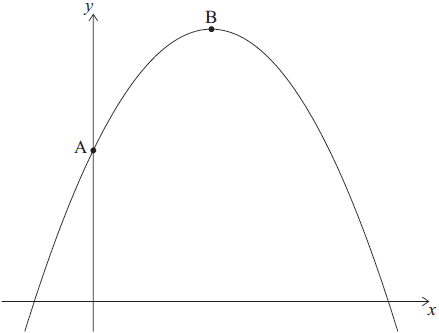Write down the value of c.

a.

Find the value of b.

b.

Find the x-intercepts of the graph of f .

c.

Write down $$f (x)$$ in the form $$f (x) = −(x − p) (x + q)$$.

d.

## Markscheme

5     (A1)     (C1)

a.

$$\frac{{ – b}}{{2( – 1)}} = 2$$     (M1)

Note: Award (M1) for correct substitution in axis of symmetry formula.

OR

$$y = 5 + bx – x^2$$

$$9 = 5 + b (2) – (2)^2$$     (M1)

Note: Award (M1) for correct substitution of 9 and 2 into their quadratic equation.

$$(b =) 4$$     (A1)(ft)     (C2)

Note: Follow through from part (a).

b.

5, −1     (A1)(ft)(A1)(ft)     (C2)

Notes: Follow through from parts (a) and (b), irrespective of working shown.

c.

$$f (x) = -(x – 5)(x + 1)$$     (A1)(ft)     (C1)

Notes: Follow through from part (c).

d.

## Question

A quadratic function $$f:x \mapsto a{x^2} + b$$, where $$a$$ and $$b \in \mathbb{R}$$ and $$x \geqslant 0$$, is represented by the mapping diagram.Using the mapping diagram, write down two equations in terms of $$a$$ and $$b$$.

a.

Solve the equations to find the value of

(i)     $$a$$;

(ii)     $$b$$.

b.

Find the value of $$c$$.

c.

## Markscheme

$$a{(1)^2} + b = – 9$$     (A1)

$$a{(3)^2} + b = 119$$     (A1)     (C2)

Note: Accept equivalent forms of the equations.[2 marks]

a.

(i)     $$a = 16$$     (A1)(ft)

(ii)     $$b = – 25$$     (A1)(ft)     (C2)

Note: Follow through from part (a) irrespective of whether working is seen.

If working is seen follow through from part (i) to part (ii).[2 marks]

b.

$$16{c^2} – 25 = 171$$     (M1)

Note: Award (M1) for correct quadratic with their $$a$$ and $$b$$ substituted.

$$c = 3.5$$     (A1)(ft)     (C2)

Note: Accept $$x$$ instead of $$c$$.

Award (A1) only, for an answer of $$\pm 3.5$$ with or without working.[2 marks]

c.

## Question

The surface of a red carpet is shown below. The dimensions of the carpet are in metres.Write down an expression for the area, $$A$$, in $${{\text{m}}^2}$$, of the carpet.

a.

The area of the carpet is $${\text{10 }}{{\text{m}}^2}$$.

Calculate the value of $$x$$.

b.

The area of the carpet is $${\text{10 }}{{\text{m}}^2}$$.

Hence, write down the value of the length and of the width of the carpet, in metres.

c.

## Markscheme

$$2x(x – 4)$$   or   $$2{x^2} – 8x$$     (A1)     (C1)

Note: Award (A0) for $$x – 4 \times 2x$$.[1 mark]

a.

$$2x(x – 4) = 10$$     (M1)

Note: Award (M1) for equating their answer in part (a) to $$10$$.

$${x^2} – 4x – 5 = 0$$     (M1)

OR

Sketch of $$y = 2{x^2} – 8x$$ and $$y = 10$$     (M1)

OR

Using GDC solver $$x = 5$$ and $$x = – 1$$     (M1)

OR

$$2(x + 1)(x – 5)$$     (M1)

$$x = 5{\text{ (m)}}$$     (A1)(ft)     (C3)

Award at most (M1)(M1)(A0) if both $$5$$ and $$-1$$ are given as final answer.

Final (A1)(ft) is awarded for choosing only the positive solution(s).[3 marks]

b.

$$2 \times 5 = 10{\text{ (m)}}$$     (A1)(ft)

$$5 – 4 = 1{\text{ (m)}}$$     (A1)(ft)     (C2)

Do not accept negative answers.[2 marks]

c.

## Question

A building company has many rectangular construction sites, of varying widths, along a road.

The area, $$A$$, of each site is given by the function

$A(x) = x(200 – x)$

where $$x$$ is the width of the site in metres and $$20 \leqslant x \leqslant 180$$.

Site S has a width of $$20$$ m. Write down the area of S.

a.

Site T has the same area as site S, but a different width. Find the width of T.

b.

When the width of the construction site is $$b$$ metres, the site has a maximum area.

(i)     Write down the value of $$b$$.

(ii)     Write down the maximum area.

c.

The range of $$A(x)$$ is $$m \leqslant A(x) \leqslant n$$.

Hence write down the value of $$m$$ and of $$n$$.

d.

## Markscheme

$$3600{\text{ (}}{{\text{m}}^2})$$     (A1)(C1)

a.

$$x(200 – x) = 3600$$     (M1)

Note: Award (M1) for setting up an equation, equating to their $$3600$$.

$$180{\text{ (m)}}$$     (A1)(ft)     (C2)

b.

(i)     $$100{\text{ (m)}}$$     (A1)     (C1)

(ii)     $$10\,000{\text{ (}}{{\text{m}}^2})$$     (A1)(ft)(C1)

$$m = 3600\;\;\;$$and$$\;\;\;n = 10\,000$$     (A1)(ft)     (C1)
Notes: Follow through from part (a) and part (c)(ii), but only if their $$m$$ is less than their $$n$$. Accept the answer $$3600 \leqslant A \leqslant 10\,000$$.